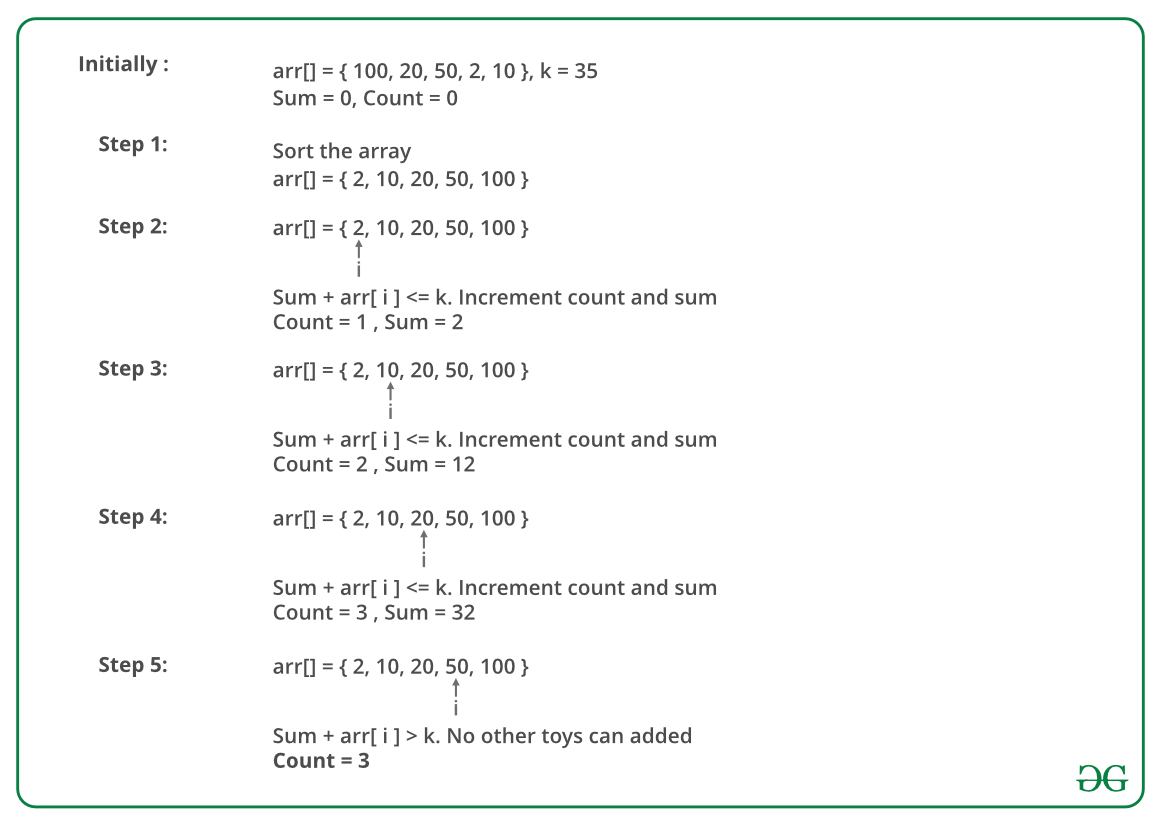Related Articles

# Maximise the number of toys that can be purchased with amount K

• Difficulty Level : Easy
• Last Updated : 27 May, 2021

Given an array consisting of the cost of toys. Given an integer K depicting the amount of money available to purchase toys. Write a program to find the maximum number of toys one can buy with the amount K.
Note: One can buy only 1 quantity of a particular toy.

Examples:

```Input:  N = 10, K =  50
cost = { 1, 12, 5, 111, 200, 1000, 10, 9, 12, 15 }
Output: 6
Explanation: Toys with amount 1, 5, 9, 10, 12, and 12
can be purchased resulting in a total amount of 49. Hence,
maximum number of toys is 6.

Input: N = 7, K = 50
cost = { 1, 12, 5, 111, 200, 1000, 10 }
Output: 4 ```

The idea to solve this problem is to first sort the cost array in ascending order. This will arrange the toys in increasing order of cost. Now iterate over the cost array and keep calculating the sum of costs until the sum is less than or equal to K. Finally, return the number of toys used to calculate the sum which is just less than or equals to K.

The image below is an illustration of the above approach:Below is the implementation of the above approach:

## C++

 `// C++ Program to maximize the``// number of toys with K amount``#include ``using` `namespace` `std;` `// This functions returns the required``// number of toys``int` `maximum_toys(``int` `cost[], ``int` `N, ``int` `K)``{``    ``int` `count = 0, sum = 0;` `    ``// sort the cost array``    ``sort(cost, cost + N);``    ``for` `(``int` `i = 0; i < N; i++) {` `        ``// Check if we can buy ith toy or not``        ``if` `(sum +cost[i] <= K)``        ``{``            ``sum = sum + cost[i];``            ``// Increment count``            ``count++;``        ``}``    ``}``    ``return` `count;``}` `// Driver Code``int` `main()``{``    ``int` `K = 50;``    ``int` `cost[] = { 1, 12, 5, 111, 200, 1000, 10, 9, 12, 15 };``    ``int` `N = ``sizeof``(cost) / ``sizeof``(cost);` `    ``cout << maximum_toys(cost, N, K) << endl;``    ``return` `0;``}`

## Java

 `// Java Program to maximize the``// number of toys with K amount``import` `java.io.*;``import` `java .util.*;` `class` `GFG``{``// This functions returns``// the required number of toys``static` `int` `maximum_toys(``int` `cost[],``                        ``int` `N, ``int` `K)``{``    ``int` `count = ``0``, sum = ``0``;` `    ``// sort the cost array``    ``Arrays.sort(cost);``    ``for` `(``int` `i = ``0``; i < N; i++)``    ``{` `        ``// Check if we can buy ith toy or not``        ``if` `(sum +cost[i] <= K)``        ``{``            ``sum = sum + cost[i];``            ``// Increment count``            ``count++;``        ``}``    ``}``    ``return` `count;``}` `// Driver Code``public` `static` `void` `main (String[] args)``{``int` `K = ``50``;``int` `cost[] = {``1``, ``12``, ``5``, ``111``, ``200``,``            ``1000``, ``10``, ``9``, ``12``, ``15``};``int` `N = cost.length;` `System.out.print( maximum_toys(cost, N, K));``}``}` `// This code is contributed by anuj_67.`

## Python3

 `# Python 3 Program to maximize the``# number of toys with K amount` `# This functions returns the required``# number of toys``def` `maximum_toys(cost, N, K):``    ``count ``=` `0``    ``sum` `=` `0` `    ``# sort the cost array``    ``cost.sort(reverse ``=` `False``)``    ``for` `i ``in` `range``(``0``, N, ``1``):``        ` `        ``# Check if we can buy ith toy or not``        ``if` `(``sum``+``cost[i] <``=` `K):``            ``sum` `=` `sum` `+` `cost[i]``            ``# Increment the count variable``            ``count ``+``=` `1``    ` `    ``return` `count` `# Driver Code``if` `__name__ ``=``=` `'__main__'``:``    ``K ``=` `50``    ``cost ``=` `[``1``, ``12``, ``5``, ``111``, ``200``,``            ``1000``, ``10``, ``9``, ``12``, ``15``]``    ``N ``=` `len``(cost)` `    ``print``(maximum_toys(cost, N, K))` `# This code is contributed by``# Sanjit_Prasad`

## C#

 `// C# Program to maximize the``// number of toys with K amount``using` `System;` `class` `GFG``{``// This functions returns``// the required number of toys``static` `int` `maximum_toys(``int` `[]cost,``                        ``int` `N, ``int` `K)``{``    ``int` `count = 0, sum = 0;` `    ``// sort the cost array``    ``Array.Sort(cost);``    ``for` `(``int` `i = 0; i < N; i++)``    ``{` `        ``// Check if we can buy ith toy or not``        ``if` `(sum +cost[i] <= K)``        ``{``            ``sum = sum + cost[i];``            ``// Increment count``            ``count++;``        ``}``    ``}``    ``return` `count;``}` `// Driver Code``public` `static` `void` `Main ()``{``int` `K = 50;``int` `[]cost = {1, 12, 5, 111, 200,``            ``1000, 10, 9, 12, 15};``int` `N = cost.Length;` `Console.Write( maximum_toys(cost, N, K));``}``}` `// This code is contributed by anuj_67.`

## PHP

 ``

## Javascript

 ``
Output :
`6`

Time Complexity: O(N * logN), where N is the size of the cost array.

Attention reader! Don’t stop learning now. Get hold of all the important DSA concepts with the DSA Self Paced Course at a student-friendly price and become industry ready.  To complete your preparation from learning a language to DS Algo and many more,  please refer Complete Interview Preparation Course.

In case you wish to attend live classes with experts, please refer DSA Live Classes for Working Professionals and Competitive Programming Live for Students.

My Personal Notes arrow_drop_up